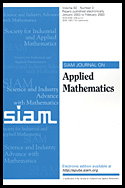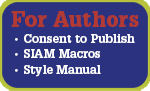## SIAM Journal on Applied Mathematics (SIAP)

Paul A. Martin, Editor-in-Chief

The SIAM Journal on Applied Mathematics is an interdisciplinary journal containing research articles that treat scientific problems using methods that are of mathematical interest. Appropriate subject areas include the physical, engineering, financial, and life sciences. Examples are problems in fluid mechanics, including reaction-diffusion problems, sedimentation, combustion, and transport theory; solid mechanics; elasticity; electromagnetic theory and optics; materials science; mathematical biology, including population dynamics, biomechanics, and physiology; linear and nonlinear wave propagation, including scattering theory and wave propagation in random media; inverse problems; nonlinear dynamics; and stochastic processes, including queueing theory. Mathematical techniques of interest include asymptotic methods, bifurcation theory, dynamical systems theory, complex network theory, computational methods, and probabilistic and statistical methods.

The SIAM Journal on Applied Mathematics is the founding journal of the Society for Industrial and Applied Mathematics.  The first volume of the journal appeared in 1953 and the foundations and evolution of applied mathematics can be found within its pages.

View this journal online